# Electronics and Communication Engineering - Exam Questions Papers

41.
The current I for the network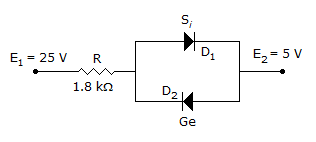0 mA
10.72 mA
10.94 mA
11.32 mA
Explanation: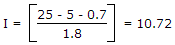.

42.
A switch tail ring counter is made by using a single D FF. The resulting circuit is a
SR FF
JK FF
D FF
T FF
Explanation: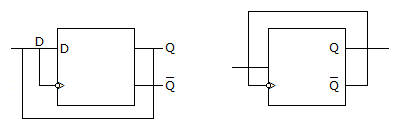In both case input will always opposite to each other hence it is D FF.

43.
The driving point impedance of the following network is given by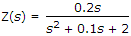. The component values are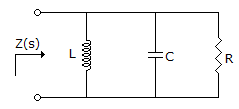L = 5H, R = 0.5Ω, C = 0.1F
L = 0.1H, R = 0.5Ω, C = 5F
L = 5H, R = 2Ω, C = 0.1F
L = 0.1H, R = 2Ω, C = 5F
Explanation: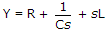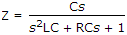Comparing it with given equation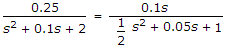C = 0.1

RC = 0.05

R = 0.54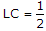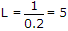.

44.
Consider a baseband binary PAM receiver shown below. The additive channel noise n(t) is whit with power spectral density SN(f) = N0/2 = 10-20 W/Hz. The low-pass filter is ideal with unity gain and cutoff frequency 1 MHz. Let Yk represent the random variable y(tk).
Yk = nNk if transmitted bit bk = 0
Yk = a + Nk if transmitted bit bk = 1
Where Nk represents the noise sample value. The noise sample has a probability density function, PNk(n) = 0.5ae-a|n| (This has mean zero and variance 2/a2). Assume transmitted bits to be equiprobable and threshold z is set to a/2 = 10-6 V.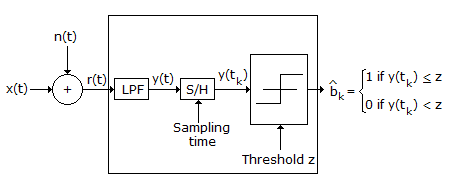The probability of bit error is
0.5 x e-3.5
0.5 x e-5
0.5 x e-7
0.5 x e-10
Explanation:

Probability of error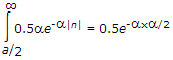= 0.5e-107 x 10 -6 = 0.5e-10.

45.
In a synchro error detector, the output voltage is proportional to [ω(t)]n, where ω(t) is the rotor velocity and n equals :
-2
-1
1
2